# 优雅的使用javascript递归画一棵结构树示例代码_凊蒝_前端开发者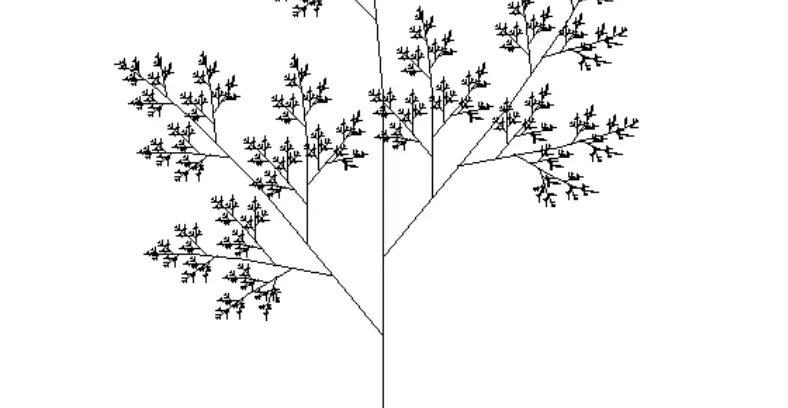```function factorial(n) {
if (n === 1) return 1;
return n * factorial(n - 1);
}

factorial(5) // 120```

```function factorial(n, total) {
return factorial(n - 1, n * total);
}

factorial(5) // 120```

1. 数组求和

```function sumArray(arr, total) {
if(arr.length === 1) {
}
return sum(arr, total + arr.pop())
}

let arr = [1,2,3,4];
sumArray(arr, arr) // 10```

2. 斐波那且数列

```// 斐波那契数列
function factorial1 (n) {
if(n <= 2){
return 1
}
return factorial1(n-1) + factorial1(n-2)
}

// 尾递归优化后
function factorial2 (n, start = 1, total = 1) {
if(n <= 2){
}
return factorial2 (n -1, total, total + start)
}```

3. 阶乘

4. 省市级联多级联动

5. 深拷贝

```function clone(target) {
if (typeof target === 'object') {
let cloneTarget = Array.isArray(target) ? [] : {};
for (const key in target) {
cloneTarget[key] = clone(target[key]);
}
return cloneTarget;
} else {
return target;
}
};```

6. 爬梯问题

```n =1; result = 1 --> 1
n =2; result = 2 --> 11 2
n =3; result = 3 --> 111 12 21
...

function steps(n) {
if(n <= 1) {
return 1
}
return steps(n-1) + steps(n-2)
}```

7. 对象数据格式化

```let obj = {
a: '1',
b: {
c: '2',
D: {
E: '3'
}
}
}

let obj = {
a: '1',
b: {
c: '2',
d: {
e: '3'
}
}
}

// 代码实现
function keysLower(obj) {
let reg = new RegExp("([A-Z]+)", "g");
for (let key in obj) {
if (obj.hasOwnProperty(key)) {
let temp = obj[key];
if (reg.test(key.toString())) {
// 将修改后的属性名重新赋值给temp，并在对象obj内添加一个转换后的属性
temp = obj[key.replace(reg, function (result) {
return result.toLowerCase()
})] = obj[key];
// 将之前大写的键属性删除
delete obj[key];
}
// 如果属性是对象或者数组，重新执行函数
if (typeof temp === 'object' || Object.prototype.toString.call(temp) === '[object Array]') {
keysLower(temp);
}
}
}
return obj;
};```

8. 遍历目录/删除目录

```function deleteFolder(path) {
var files = [];
if(fs.existsSync(path)) { // 如果目录存在
files.forEach(function(file,index){
var curPath = path + "/" + file;
if(fs.statSync(curPath).isDirectory()) { // 如果是目录，则递归
deleteFolder(curPath);
} else { // 删除文件
}
});
fs.rmdirSync(path);
}
}```

9. 绘制分形图形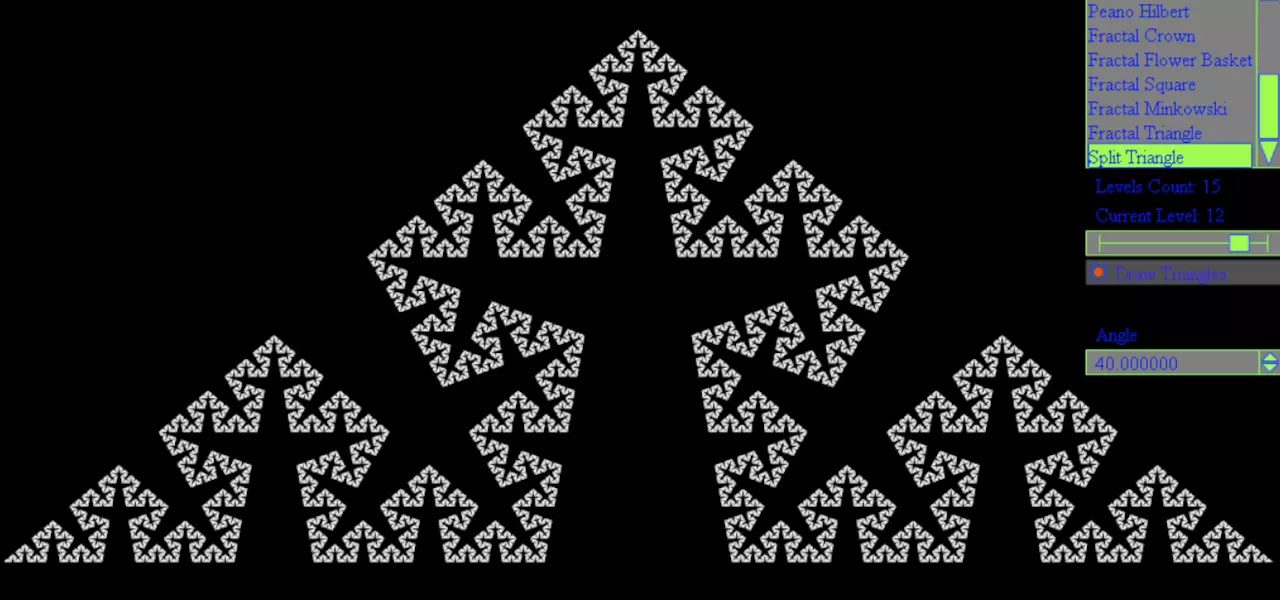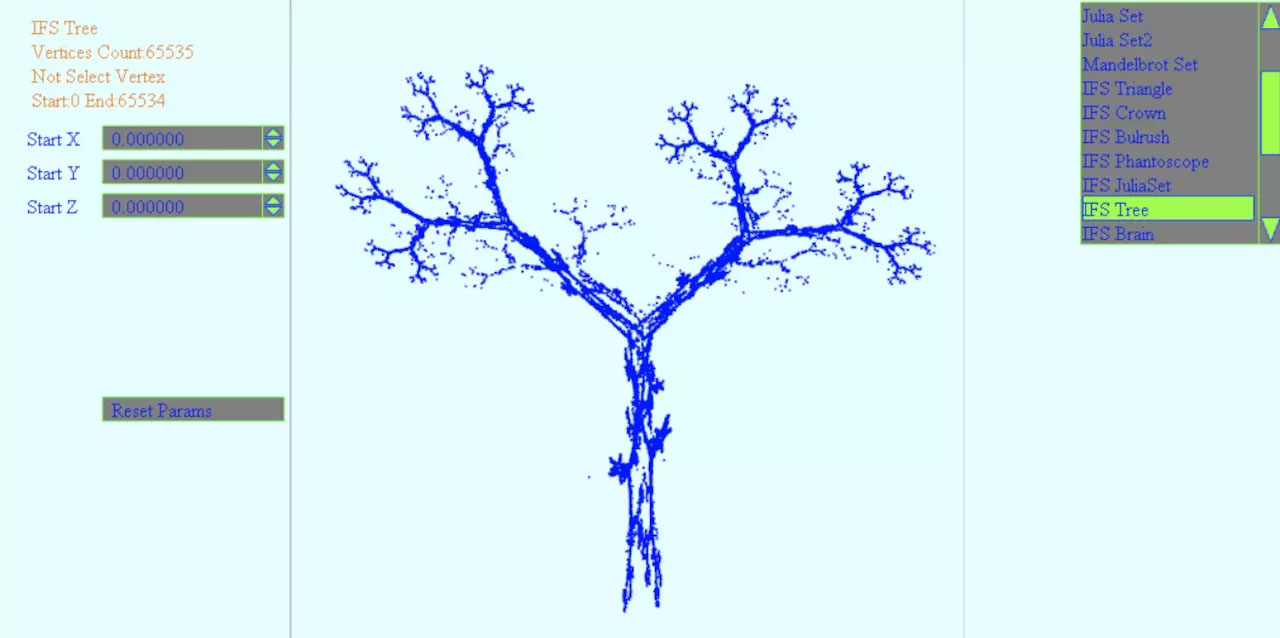10. 扁平化数组Flat

```let a = [1,2,3, [1,2,3, [1,2,3]]]
// 变成
let a = [1,2,3,1,2,3,1,2,3]
// 具体实现
function flat(arr = [], result = []) {
arr.forEach(v => {
if(Array.isArray(v)) {
result = result.concat(flat(v, []))
}else {
result.push(v)
}
})
return result
}

flat(a)```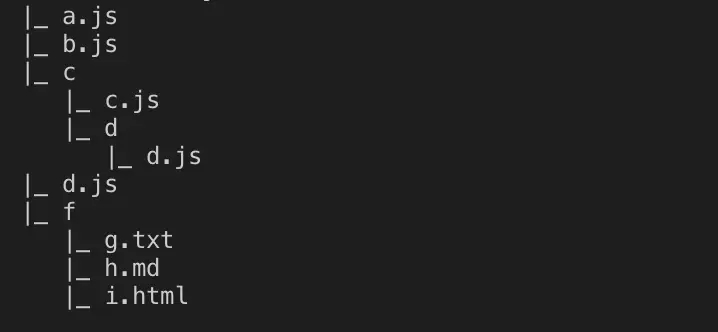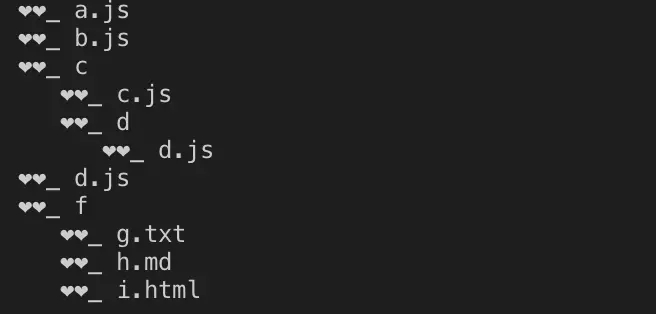```const fs = require('fs')
const path = require('path')
// 遍历目录/生成目录树
function treeFolder(path, flag = '|_') {
var files = [];

if(fs.existsSync(path)) {
files.forEach(function(file,index){
var curPath = path + "/" + file;
if(fs.statSync(curPath).isDirectory()) { // recurse
// obj[file] = treeFolder(curPath, {});
console.log(flag, file)
treeFolder(curPath, '  ' + flag)
} else {
// obj['--'] = file
console.log(flag, file)
}
})
// return obj
}
}

treeFolder(path.resolve(__dirname, './test'))```

test为我们建的测试目录，如下：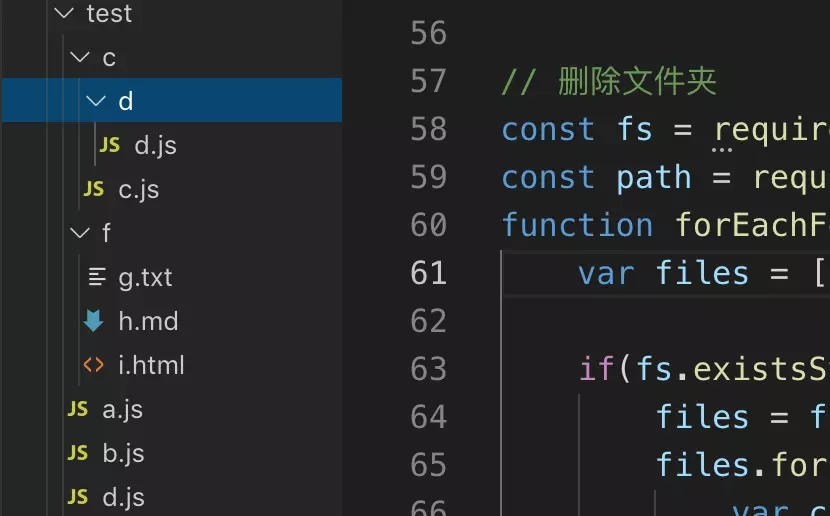64K